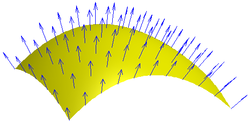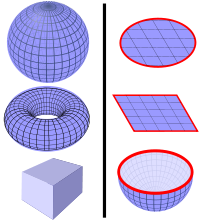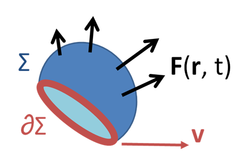# Magnetic flux

Magnetic flux

Magnetic flux (most often denoted as Φm), is a measure of the amount of magnetic B field (also called "magnetic flux density") passing through a given surface (such as a conducting coil). The SI unit of magnetic flux is the weber (Wb) (in derived units: volt-seconds). The CGS unit is the maxwell.

## DescriptionFigure 1: The definition of surface integral relies on splitting the surface into small surface elements. Each element is associated with a vector dS of magnitude equal to the area of the element and with direction normal to the element and pointing outward.Figure 2: A vector field of normals to a surface

The magnetic flux through a given surface is proportional to the number of magnetic B field lines that pass through the surface. This is the net number, i.e. the number passing through in one direction, minus the number passing through in the other direction. (See below for how the positive sign is chosen.) For a uniform magnetic field B passing through a perpendicular area the magnetic flux is given by the product of the magnetic field and the area element. The magnetic flux for a uniform B at any angle to a surface is defined by a dot product of the magnetic field and the area element vector a.$\displaystyle \Phi_m = \mathbf{B} \cdot \mathbf{a} = Ba \cos \theta$    (uniform B with flat area only)

where θ is the angle between B and a vector a that is perpendicular (normal) to the surface.

In the general case, the magnetic flux through a surface S is defined as the integral of the magnetic field over the area of the surface (See Figures 1 and 2):$\Phi_m = \iint\limits_S \mathbf{B} \cdot d\mathbf S,$

where$\textstyle \Phi_m \$ is the magnetic flux, B is the magnetic field,

S is the surface (area),$\cdot$ denotes dot product, and dS is an infinitesimal vector, whose magnitude is the area of a differential element of S, and whose direction is the surface normal. (See surface integral for more details.)

From the definition of the magnetic vector potential A and the fundamental theorem of the curl the magnetic flux may also be defined as:$\Phi_m = \oint\limits_{\Sigma} \mathbf{A} \cdot d\boldsymbol{\ell}$

where the closed line integral is over the boundary of the surface and d is an infinitesimal vector element of that contour Σ.

The magnetic flux is usually measured with a fluxmeter. The fluxmeter contains measuring coils and electronics that evaluates the change of voltage in the measuring coils to calculate the magnetic flux.

## Magnetic flux through a closed surfaceSome examples of closed surfaces (left) and open surfaces (right). Left: Surface of a sphere, surface of a torus, surface of a cube. Right: Disk surface, square surface, surface of a hemisphere. (The surface is blue, the boundary is red.)

Gauss's law for magnetism, which is one of the four Maxwell's equations, states that the total magnetic flux through a closed surface is equal to zero. (A "closed surface" is a surface that completely encloses a volume(s) with no holes.) This law is a consequence of the empirical observation that magnetic monopoles have never been found.

In other words, Gauss's law for magnetism is the statement:$\Phi_m=\int \!\!\! \int \mathbf{B} \cdot d\mathbf S = 0,$

for any closed surface S.

## Magnetic flux through an open surfaceFigure 3: A vector field F ( r, t ) defined throughout space, and a surface Σ bounded by curve ∂Σ moving with velocity v over which the field is integrated.

While the magnetic flux through a closed surface is always zero, the magnetic flux through an open surface need not be zero and is an important quantity in electromagnetism. For example, a change in the magnetic flux passing through a loop of conductive wire will cause an electromotive force, and therefore an electric current, in the loop. The relationship is given by Faraday's law:$\mathcal{E} = \oint_{\partial \Sigma (t)}\left( \mathbf{E}( \mathbf{r},\ t) +\mathbf{ v \times B}(\mathbf{r},\ t)\right) \cdot d\boldsymbol{\ell} = -{d\Phi_m \over dt},$

where (see Figure 3):$\mathcal{E}$ is the EMF,
Φm is the flux through a surface with an opening bounded by a curve ∂Σ(t),
∂Σ(t) is a closed contour that can change with time; the EMF is found around this contour, and the contour is a boundary of the surface over which Φm is found,
d is an infinitesimal vector element of the contour ∂Σ(t),
v is the velocity of the segment d,
E is the electric field,
B is the magnetic field.

The EMF is determined in this equation in two ways: first, as the work per unit charge done against the Lorentz force in moving a test charge around the (possibly moving) closed curve ∂Σ(t), and second, as the magnetic flux through the open surface Σ(t).

This equation is the principle behind an electrical generator.

## Comparison with electric flux

By way of contrast, Gauss's law for electric fields, another of Maxwell's equations, is$\Phi_E = \int \!\!\!\int_S \mathbf{E}\cdot d\mathbf{S} = {Q \over \epsilon_0},$

where

E is the electric field,
S is any closed surface,
Q is the total electric charge inside the surface S,
ε0 is the electric constant (a universal constant, also called the "permittivity of free space").

Note that the flux of E through a closed surface is not always zero; this indicates the presence of electric "monopoles", that is, free positive or negative charges.

Magnetic Circuits

Conventional Magnetic Circuits
• Magnetomotive force$\mathcal F$
• Magnetic flux Φ
• Magnetic reluctance$\mathcal R$

Phasor Magnetic Circuits

Related Concepts

Gyrator-capacitor model variables
This box:

• Magnetic field
• Maxwell's equations (sometimes called the Maxwell equations) are the set of four equations, attributed to James Clerk Maxwell, that describe the behavior of both the electric and magnetic fields, as well as their interactions with matter.
• Gauss's law gives the relation between the electric flux flowing out a closed surface and the electric charge enclosed in the surface.
• Magnetic circuit is a method using an analogy with electric circuits to calculate the flux of complex systems of magnetic components.
• Magnetic monopole is a hypothetical particle that may be loosely described as "a magnet with only one pole".
• Magnetic flux quantum is the quantum of magnetic flux passing through a superconductor.
• Carl Friedrich Gauss developed a fruitful collaboration with the physics professor Wilhelm Weber; it led to new knowledge in the field of magnetism.
• James Clerk Maxwell demonstrated that electric and magnetic forces are two complementary aspects of electromagnetism.

## External articles

Patents

Wikimedia Foundation. 2010.

### Look at other dictionaries:

• magnetic flux — n. 1. A measure of the strength of a magnetic field over a given area. [WordNet 1.5] 2. Same as {magnetic field}. [WordNet 1.5] …   The Collaborative International Dictionary of English

• magnetic flux — magnetic field magnetic field n. (Physics) The space around a magnet through which it exerts magnetic force; a field of force surrounding a permanent magnet, electrical current, or a moving charged particle; called also {magnetic flux} and {field …   The Collaborative International Dictionary of English

• magnetic flux — n. the component of a magnetic field perpendicular to an area multiplied by the size of that area; a measure of the number of magnetic field lines passing through the area: it is measured in maxwells or webers: symbol, Φ …   English World dictionary

• magnetic flux — noun 1. a measure of the strength of a magnetic field over a given area • Hypernyms: ↑magnetization, ↑magnetisation • Hyponyms: ↑flux unit, ↑magnetic flux unit 2. the lines of force surrounding a permanent magnet or a moving charged particle • …   Useful english dictionary

• magnetic flux — magnetinis srautas statusas T sritis Standartizacija ir metrologija apibrėžtis Apibrėžtį žr. priede. priedas( ai) Grafinis formatas atitikmenys: angl. magnetic flow; magnetic flux vok. Magnetfluss, m; magnetischer Fluss, m rus. магнитный поток, m …   Penkiakalbis aiškinamasis metrologijos terminų žodynas

• magnetic flux — magnetinis srautas statusas T sritis fizika atitikmenys: angl. magnetic flow; magnetic flux vok. Magnetfluß, m; magnetischer Fluß, m rus. магнитный поток, m pranc. flux magnétique, m …   Fizikos terminų žodynas

• magnetic flux — (Φ) a quantitative measure of a magnetic field, equal to the integral over a specified surface of the magnetic flux density perpendicular to the surface …   Medical dictionary

• magnetic flux — /mægˌnɛtɪk ˈflʌks/ (say mag.netik fluks) noun 1. a measure of the strength of a magnetic field, equal to the product of the average magnetic flux density and the perpendicular area through which it penetrates; the derived SI unit is the weber. 2 …

• magnetic flux — magnetinis srautas statusas T sritis automatika atitikmenys: angl. magnetic flux vok. magnetischer Fluß, m rus. магнитный поток, m pranc. flux magnétique, m …   Automatikos terminų žodynas

• Magnetic flux leakage — (MFL) is a magnetic method of nondestructive testing that is used to detect corrosion and pitting in steel structures, most commonly pipelines and storage tanks. The basic principle is that a powerful magnet is used to magnetize the steel. At… …   Wikipedia